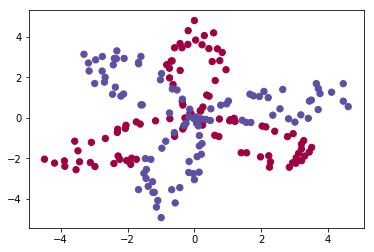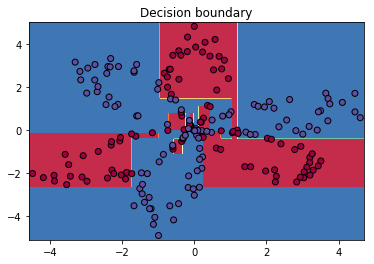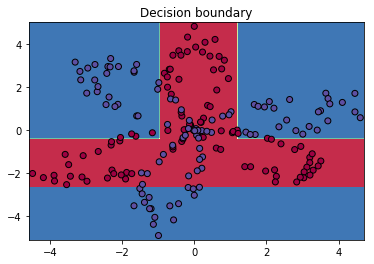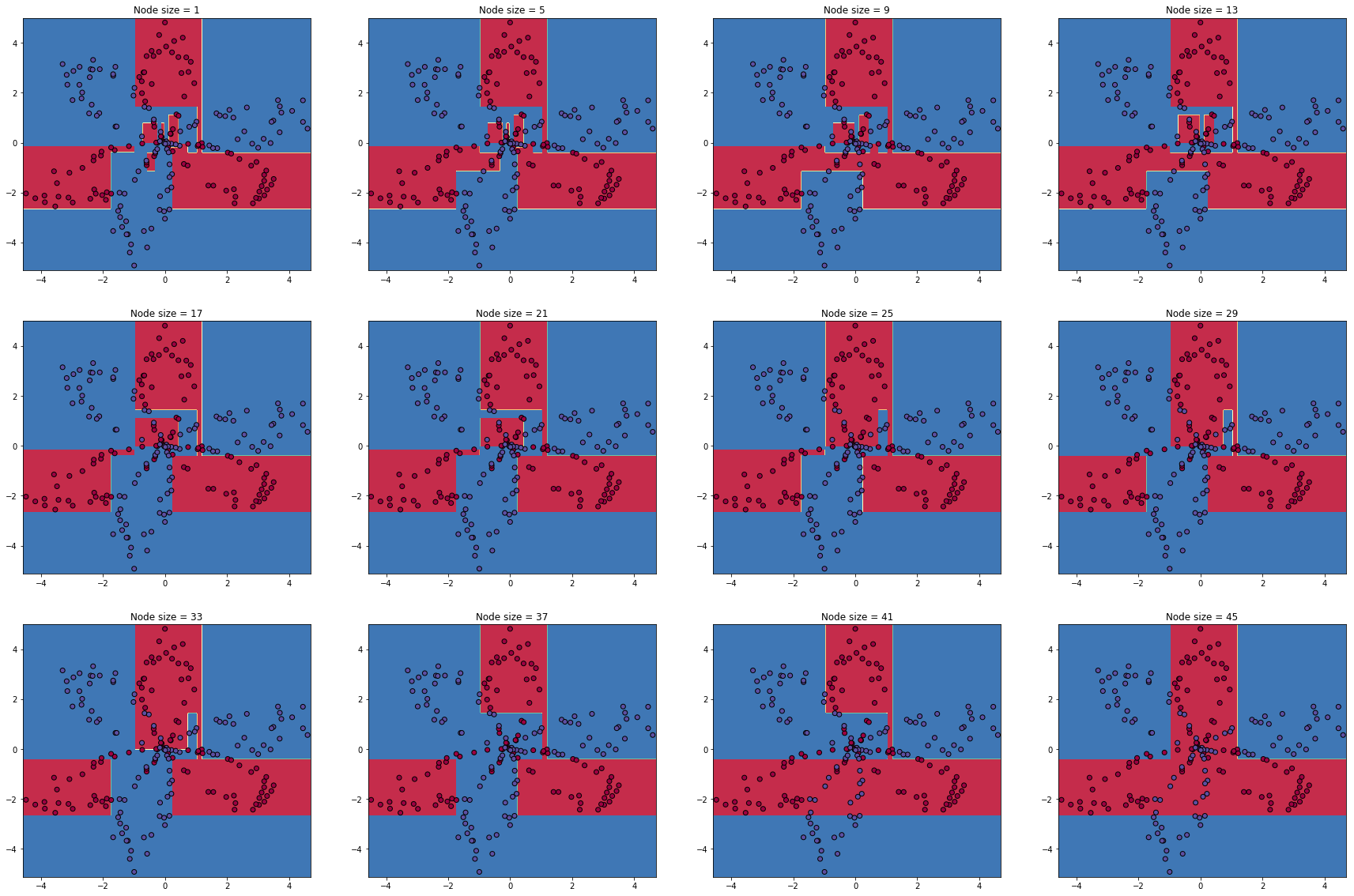# Decision Trees¶

Nov. 29, 2018 $\newcommand{\bs}{\boldsymbol}$ $\newcommand{\argmin}{\underset{\bs{#1}}{\text{arg min}}\,}$ $\newcommand{\argmax}{\underset{\bs{#1}}{\text{arg max}}\,}$ $\newcommand{\tr}{^{\top}}$ $\newcommand{\norm}{\left|\left|\,#1\,\right|\right|}$ $\newcommand{\given}{\,|\,}$

Decision trees is one of the most powerful learning algorithms. Today we will be using Python to build a decision tree from scratch. First let's load a flower dataset that we will be classifying.

In :
import numpy as np
import matplotlib.pyplot as plt
from scipy import stats

In :
def load_data():

np.random.seed(1)
m = 200 # number of examples
N = int(m/2) # number of points per class
X = np.zeros((m,2)) # data matrix where each row is a single example
Y = np.zeros(m, dtype='uint8') # labels vector (0 for red, 1 for blue)

for j in range(2):
ix = range(N*j,N*(j+1))
t = np.linspace(0, 2*3.12, N) # theta
r = 4*np.cos(3*(t+j*3.14/4)) + np.random.randn(N)*0.5 # radius
X[ix] = np.c_[r*np.sin(t), r*np.cos(t)]
Y[ix] = j

return X, Y

In :
# load and plot data
plt.scatter(X[:,0], X[:,1], c=y, s=40, cmap=plt.cm.Spectral)
plt.show()The data consists of two flowers. They are generated according to the polar equations

$$r_1 = 4\cos3\theta \,\, \text{ and } \,\, r_2 = 4\cos 3(\theta+\pi/4)$$

Clearly linear model will fail here badly. But classification trees have the flexibility to learn this data.

## Regression Trees¶

The idea behind tree-based methods is simple: We recursively split the feature space into two "rectangular" regions based on a cut-off point of one particular feature, and fit a constant model to each rectangle. First, let's consider a regression problem with continuous output variable $Y$, and $p$-dimensional input variable $\bs{X} = (X_1, ..., X_p)'$. Given a set of training examples $(\bs{x}^{(i)}, y^{(i)})$ for $i=1,2,..., N$, the algorithm needs to decide how to split the feature space into such rectangles. Suppose that this job is accomplished and we have $M$ regions $R_1, R_2,..., R_M$. Our regression model becomes

$$f(\bs{x}) = \sum_{m=1}^M c_m I(\bs{x} \in R_m),$$

where $I(\cdot)$ is the usual indicator function, and $c_m$ is a constant equal to the average of response variable $Y$ in the region $R_m$. If we can find the best binary partition that minimizes the RSS, $\sum (y^{(i)} - f(\bs{x}^{(i)}))^2$, then we will be done. However, such minimization is computationally infeasible since the number of possible partitions is unimaginably large. Instead, we use the following greedy scheme.

Choose some $j$th input variable $X_j$ ($0\leq j \leq p$) and a split point $s$. Define the pair of half-planes

$$R_1(j, s) = \{\bs{X} \given X_j\leq s\} \,\, \text{ and } \,\, R_2(j,s)=\{\bs{X}\given X_j >s\}. \tag{1}$$

Each binary split into two rectangles can be viewed as a splitting end of a tree branch. The splitting processes are also called the nodes of the tree, and any terminal node where there is no more splitting is called a leaf. The feature variable $X_j$ and the split point $s$ are chosen to minimizes the total RSS:

$$\sum_{\bs{x}^{(i)}\in R_1(j,s)} (y^{(i)} - c_1)^2 + \sum_{\bs{x}^{(i)} \in R_2(j,s)} (y^{(i)}-c_2)^2. \tag{2}$$

This problem is quick to solve computationally via enumeration. After we find the optimal split, we repeat the process to each of the two resulting regions. The next question we should ask is:

When should we stop the splitting process?

The best procedure is to first grow the tree as large as possible (stop only when some minimum node size, say 5, is reached). Then, we prune the tree using the following procedure.

## Pruning¶

Let $T_0$ be the original tree and let $T \subset T_0$ denote the pruned subtree. The pruning is done by reversing the splitting processes in (1). This is exactly analogous to pruning a real tree without breaking its main branch. Let $|T|$ denote the number of leaves in $T$. Let $N_m$ denote the number of training examples in the $m$th leaf $R_m$, where $1\leq m \leq |T|$. We define the cost complexity of the subtree $T$ as

$$C_{\alpha}(T) = \sum_{m=1}^{|T|} \sum_{\bs{x}^{(i)} \in R_m} (y^{(i)} - c_m)^2 + \alpha|T|. \tag{3}$$

where again $c_m$ is the average of the response variable $Y$ for all training examples in $R_m$, and can be written formally as $c_m = \text{ave}(y^{(i)} \given \bs{x}^{(i)} \in R_m)$. The pruning parameter $\alpha \geq 0$ imposes a penalty for large tree sizes, and the degree of pruning depends on the magnitude of $\alpha$. Clearly, when $\alpha =0$, we get the original tree $T_0$ without pruning. So the remaining task is to choose the parameter $\alpha$.

For each value of $\alpha$, there is a unique subtree $T_{\alpha}$ that minimizes the cost complexity $C_{\alpha}(T)$. To find $T_{\alpha}$ we use a method called cost complexity pruning. We successively collapse the interval node of the tree that produces the smallest increase in the cost complexity defined in (2). The process ends until the tree becomes a single stump. Among this finite sequence of subtrees, we can find $T_{\alpha}$. Finally, using a whole range of values of $\alpha$, we can use the K-fold cross-validation technique to choose the best $\alpha$.

## Classification Trees¶

A classification tree is very similar to a regression tree, except that we can no longer use the RSS term in (2). Suppose the response variable $Y$ takes values $1,2,..., K$. In the terminal rectangle $R_m$, we define the proportion of class $k$ to be

$$\widehat{p}_{mk} = \text{ave}\left(I(y^{(i)} = k) \given \bs{x}^{(i)} \in R_m \right).$$

Unlike in regression trees where we classify the observations by taking the average value of responses in a rectangle, the classification tree assigns an observation in $R_m$ to the majority class $k$ in $R_m$, which is the class $k$ that maximizes $\widehat{p}_{mk}$. Despite the fixed classification of each terminal rectangle, we could interpret the process as classifying to class $k$ with probability $\widehat{p}_{mk}$. Additionally, we could use one-vs-all encoding by letting 1 represent class $k$ and 0 for all other classes. Then the variance over the training examples in $R_m$ is simply the binomial variance $\widehat{p}_{mk} (1-\widehat{p}_{mk})$. Summing over the variances across all terminal nodes, we obtain a impurity measure called the Gini index, defined as

$$G_m = \sum_{k=1}^K \widehat{p}_{mk} ( 1-\widehat{p}_{mk}).$$

Using the same split scheme as in (1), at each iteration, we choose a feature variable $X_j$ and a split point $s$ that minimizes the weighted impurity measure:

$$w_1 G_1 + w_2 G_2. \tag{4}$$

where $w_i$ is the weight associated to $R_i(j,s)$, obtained by dividing the number of training examples by the total number of training examples before the split, and $G_i$ is the Gini index for $R_i(j,s)$, for $i=1,2$. (Note that we could also use $D_i$.)

Using the Gini index, the cost-complexity in (3) is modified to be

$$C_{\alpha}(T) = \sum_{m=1}^{|T|} w_m G_m + \alpha|T|. \tag{5}$$

where $w_m$ is weight assigned to the $m$th leaf $R_m$, which is proportional to the number of training examples in $R_m$.

## Implementation¶

We will build a classification tree from scratch. First let's define the Gini index using vectorized implementation in numpy.

In :
def Gini(y):

"""y is a vector of class labels"""

k = len(np.unique(y)) # number of unique classes
p = np.zeros(k) # class proportions [p1,...,pk] in R

for i in range(k):
p[i] = np.mean(i == y)

return np.dot(p, (1-p))

In :
# test 1
y1 = np.array([1,1,1,2,2,2,3])
print(Gini(y1))

0.48979591836734687

In :
# test 2
y2 = np.array([1,1,1,1,1,1,1])
print(Gini(y2))

0.0


Next we will define each node in the decision as a Python class. The class serves as a dictionary that holds the values of data and label in each node in the tree. The reason I use class instead of dictionary is that I can add on different method to it. For example, within each node, I can give predictions based on the majority rule.

In :
class node():

"""node containing data and label"""

def __init__(self, X, y):
self.data = X
self.label = y

def prediction(self): # make prediction
return stats.mode(self.label)

def score(self): # return probability for prediction
return round(stats.mode(self.label)/len(self.label), 2)

In :
# Let's build a mini data set
np.random.seed(1)
data = np.random.randn(20, 2)
label = np.random.randint(0, 3, 20)

# print first 5 rows of dataset
import pandas as pd
df = pd.DataFrame(data, columns=['X1', 'X2'])
df['Y'] = label

         X1        X2  Y
0  1.624345 -0.611756  0
1 -0.528172 -1.072969  1
2  0.865408 -2.301539  1
3  1.744812 -0.761207  1
4  0.319039 -0.249370  0

In :
testnode = node(data, label)
print('Prediction:', testnode.prediction())
print('Probability:', testnode.score())

Prediction: 0
Probability: 0.4


Next we will define a split function that splits the feature space by minimizing the weighted impurity measure defined in (4).

In :
def split(X, y, j, s):
'''
A split on variable j and point s

**Parameters**
X = data matrix with dimension (n x p)
y = class labels of length n
j = feature number
s = split point on feature j
'''
Xj = X[:,j]
return X[Xj > s], X[Xj <= s], y[Xj > s], y[Xj <= s]

In :
def best_split(R, min_node_size=1):
'''
Find the best split

**Parameters**
R = node class containing data and label
min_node_size = minimum size of the node for split
'''
X, y = R.data, R.label # reading data and label from node

if len(y) <= min_node_size:
return node(X,y), None, None, None # no change
else:
impurity, impurity_index = [], []

for j in range(X.shape): # choose feature j
for s in X[:,j]: # choose split point s
X1, X2, y1, y2 = split(X, y, j, s)
w = X1.shape/(X1.shape+X2.shape) # calculate weights
impurity.append(w*Gini(y1) + (1-w)*Gini(y2)) # saving impurity
impurity_index.append((j,s)) # saving feature and split point

impurity_min = min(impurity) # minimum impurity value

if impurity_min >= Gini(y):
return node(X,y), None, None, None # no change
else:
best_index = impurity.index(impurity_min) # find min index
(j,s) = impurity_index[best_index] # obtain best (j,s)
X1, X2, y1, y2 = split(X, y, j, s) # find the best split
return node(X1, y1), node(X2, y2), j, s

In :
# test
print(best_split(testnode))

<__main__.node object at 0x7f57a92c5cf8>


Next, we create a class that asks questions that divide the feature space.

In :
class question:

"""Used for printing tree and making predictions"""

def __init__(self, j, s):
self.feature = j # feature number
self.cut_off = s # split point

# determine whether example belongs to the right split
def match(self, example):
val = example[self.feature]
return val >= self.cut_off

# Print the question
def __repr__(self):
return "Is %s %s %s?" % (
"Var " + str(self.feature+1), ">=", str(round(self.cut_off, 2)) )


In :
# test
myquestion = question(0, 1)
print(myquestion)

Is Var 1 >= 1?

In :
# test
print(myquestion.match([0, 1, 2]), ';', myquestion.match([2, 1, 2]))

False ; True


Now we are ready to build the tree using recursive programming.

In :
def build_tree(R, min_node_size=1, verbose=True):

"""R is the initial node in the tree"""

R1, R2, j, s = best_split(R, min_node_size) # do a split

# When there is no more split, a leaf is formed.
if (R2 == None):
if verbose:
print("A leaf has formed.")
return R1

# next we resursively split the tree
if verbose:
print("Split occured on Var {} at {}".format(j, s))
R11 = build_tree(R1, min_node_size, verbose)
R22 = build_tree(R2, min_node_size, verbose)
return {"left_split": R11, "right_split": R22, "question": question(j,s)}

In :
# test
mytesttree = build_tree(testnode, min_node_size=5)

Split occured on Var 0 at -0.2678880796260159
Split occured on Var 1 at -0.7612069008951028
Split occured on Var 0 at 0.31903909605709857
A leaf has formed.
A leaf has formed.
Split occured on Var 0 at -0.12289022551864817
A leaf has formed.
A leaf has formed.
Split occured on Var 0 at -0.671246130836819
A leaf has formed.
A leaf has formed.


But this is not the best representation of a tree. It helps to print out the structure of a tree.

In :
def print_tree(mytree, spacing=""):

"""mytree is the output of build_tree function"""

# final step of recursion; we have reached a leaf
if isinstance(mytree, node):
print(spacing + "---------------------------")
print (spacing + "Predict:", mytree.prediction(), "- ( Prob:", mytree.score(), ")")
print(spacing + "---------------------------")
return

# Print the question at this node
print (spacing + str(mytree["question"]))

# recursively call the function on the first split
print (spacing + '--> True:')
print_tree(mytree["left_split"], spacing + "    ")

# recursively call the function on the second split
print (spacing + '--> False:')
print_tree(mytree["right_split"], spacing + "    ")


Let's print our tree!

In :
print_tree(mytesttree)

Is Var 1 >= -0.27?
--> True:
Is Var 2 >= -0.76?
--> True:
Is Var 1 >= 0.32?
--> True:
---------------------------
Predict: 2 - ( Prob: 0.75 )
---------------------------
--> False:
---------------------------
Predict: 0 - ( Prob: 1.0 )
---------------------------
--> False:
Is Var 1 >= -0.12?
--> True:
---------------------------
Predict: 1 - ( Prob: 0.75 )
---------------------------
--> False:
---------------------------
Predict: 0 - ( Prob: 0.5 )
---------------------------
--> False:
Is Var 1 >= -0.67?
--> True:
---------------------------
Predict: 1 - ( Prob: 1.0 )
---------------------------
--> False:
---------------------------
Predict: 0 - ( Prob: 0.6 )
---------------------------


This is precisely why tree is one of the best classification algorithms in machine learning. The interpretability cannot be beat! All one needs to do is to follow a series of written questions to obtain the final result. Next we define a function that make classification based on a single training data.

In :
# define a function that can classify a single training example
def classify(mydata, mytree):

"""mydata is a p-dimensional vector (x1,...,xp)"""

# reaching a leaf, make prediction!
if isinstance(mytree, node):
return mytree.prediction()

# recursively classify the two split data sets
if mytree["question"].match(mydata):
return classify(mydata, mytree["left_split"])
else:
return classify(mydata, mytree["right_split"])

In :
# test
print(classify([1, 2], mytesttree), ';', classify([0, 1], mytesttree))

2 ; 0

In :
# extend to a set of training examples
def classify_batch(mydata, mytree):

"""mydata is a matrix of dimension (m, p)"""

predictions = []

# make a prediction for each row of the matrix
for i in range(mydata.shape):
predictions.append(classify(mydata[i], mytree))

return np.array(predictions)

In :
# test
print(classify_batch(np.array([[1,2],[0,1]]), mytesttree))

[2 0]


Now we are ready to classify the original flower dataset.

In :
mynode = node(X,y) # initialize the node
mytree = build_tree(mynode, min_node_size=1, verbose=False) # build a tree


Let's visualize the decision boundary of the simple (unpruned) classification that we built.

In :
# Define a function that plots decision boundary of 2D classification problem
def plot_decision_boundary_tree(mytree, data, label):

# plt.figure(figsize=[10,10])
x_min, x_max = data[:, 0].min()-0.1, data[:, 0].max()+0.1
y_min, y_max = data[:, 1].min()-0.2, data[:, 1].max()+0.2
xx, yy = np.meshgrid(np.arange(x_min, x_max, 0.02), np.arange(y_min, y_max, 0.02))
Z = classify_batch(np.c_[xx.ravel(), yy.ravel()], mytree)

# Use a contour plot to show the decision boundary
Z = Z.reshape(xx.shape)
plt.contourf(xx, yy, Z, cmap=plt.cm.Spectral)

# Plot also the training points
plt.scatter(data[:, 0], data[:, 1], c=label, cmap=plt.cm.Spectral, edgecolors='k')
plt.title('Decision boundary')

In :
plot_decision_boundary_tree(mytree, X, y)Well, the decision boundary looks "boxy", and certainly needs some improvement. But overall, for such a simple model, this is doing great! We can increase the minimum node size to 20.

In :
# build a tree
mytree2 = build_tree(mynode, min_node_size=50, verbose=False)
# print tree
print_tree(mytree2)

Is Var 2 >= -2.68?
--> True:
Is Var 2 >= -0.4?
--> True:
Is Var 1 >= 1.22?
--> True:
---------------------------
Predict: 1 - ( Prob: 1.0 )
---------------------------
--> False:
Is Var 1 >= -0.98?
--> True:
Is Var 2 >= 1.43?
--> True:
---------------------------
Predict: 0 - ( Prob: 1.0 )
---------------------------
--> False:
---------------------------
Predict: 0 - ( Prob: 0.5 )
---------------------------
--> False:
---------------------------
Predict: 1 - ( Prob: 0.86 )
---------------------------
--> False:
Is Var 1 >= 0.23?
--> True:
---------------------------
Predict: 0 - ( Prob: 1.0 )
---------------------------
--> False:
---------------------------
Predict: 0 - ( Prob: 0.68 )
---------------------------
--> False:
---------------------------
Predict: 1 - ( Prob: 1.0 )
---------------------------

In :
plot_decision_boundary_tree(mytree2, X, y)So leaf size controls the degree of over-fitting. We will plot the decision boundary for various classification minimum leaf sizes.

In :
# Define a function that plots decision boundary of 2D classification problem
def plot_decision_boundary_object(mytree, data, label):
x_min, x_max = data[:, 0].min()-0.1, data[:, 0].max()+0.1
y_min, y_max = data[:, 1].min()-0.2, data[:, 1].max()+0.2
xx, yy = np.meshgrid(np.arange(x_min, x_max, 0.02), np.arange(y_min, y_max, 0.02))
Z = classify_batch(np.c_[xx.ravel(), yy.ravel()], mytree)
# Use a contour plot to show the decision boundary
Z = Z.reshape(xx.shape)
return xx, yy, Z

f, ax = plt.subplots(3,4, figsize=(30,20))

for i in range(12):
mytree = build_tree(mynode, min_node_size=1+4*i, verbose=False)
xx, yy, Z = plot_decision_boundary_object(mytree, X, y)
ax[i//4, i%4].contourf(xx, yy, Z, cmap=plt.cm.Spectral)
ax[i//4, i%4].scatter(X[:,0], X[:,1], c=y, cmap=plt.cm.Spectral, edgecolors='k')
ax[i//4, i%4].set_title("Node size = " + str(4*i+1))
plt.show()